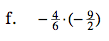Home > CC2 > Chapter 8 > Lesson 8.2.2 > Problem8-48

8-48.
1. Simplify each expression. Homework Help ✎

1.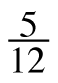+ (−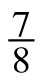) − (−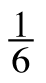)

2.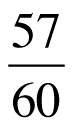3. 4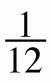+ (−1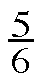)

4.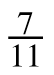·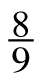5.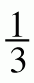·+6.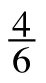· (−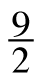)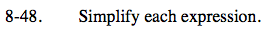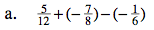Find a common denominator.

$-\frac{7}{24}$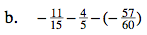Find a common denominator.

$-\frac{44}{60}-\frac{48}{60}-\left(-\frac{57}{60}\right)$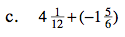Convert these mixed numbers into fractions greater than one.

$\frac{49}{12}-\frac{11}{6}$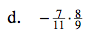$-\frac{56}{99}$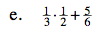Follow the Order of Operations.

$\frac{1}{6}+\frac{5}{6}$